## How to prepare for IIT JAM Physics

Joint Admission Test(JAM) is an all India admission test conducted jointly by the Indian Institute of Technology(IITs). This exam is to get admission in IITs for M.Sc.,integrated Phd and M.Sc.-Phd dual degree. It was first conducted in 2004. Every year approximately 10,000-11,000 students appear for Physics in this exam. Precisely defined syllabus of this exam makes it easier to qualify the cut off, but for better result or to get admission into IITs you need good score. Here in this post I am going to discuss on "How to prepare Physics for IIT JAM".
So let us have a look on syllabus which makes many things clear that what to read and what to leave.

### Syllabus of physics for IIT JAM

 Mathematical Methods: Calculus of single and multiple variables, partial derivatives, Jacobian,  imperfect and perfect differentials, Taylor expansion, Fourier series. Vector algebra, Vector Calculus, Multiple integrals, Divergence theorem, Green’s theorem, Stokes’ theorem. First order equations and linear second order differential equations with constant coefficients. Matrices and determinants, Algebra of complex numbers. Mechanics and General Properties of Matter: Newton’s laws of motion and applications, Velocity and acceleration in Cartesian, polar and cylindrical coordinate systems, uniformly rotating frame, centrifugal and Coriolis forces, Motion under a central force, Kepler’s laws, Gravitational Law and field, Conservative and non-conservative forces. System of particles, Center of mass, equation of motion of the CM, conservation of linear and angular momentum, con-servation of energy, variable mass systems. Elastic and inelastic collisions. Rigid body motion, fixed axis rotations, rotation and translation, moments of Inertia and products of Inertia, parallel and perpendicular axes theorem. Principal moments and axes. Kinematics of moving fluids, equation of continuity, Euler’s equation, Bernoulli’s theorem. Oscillations, Waves and Optics: Differential equation for simple harmonic oscillator and its general solution. Superposition of two or more simple harmonic oscillators. Lissajous figures. Damped and forced oscillators, resonance. Wave equation, traveling and standing waves in onedimension. Energy density and energy transmission in waves. Group velocity and phase velocity. Sound waves in media. Doppler Effect. Fermat’s Principle. General theory of image formation. Thick lens, thin lens and lens combinations. Interference of light, optical path retardation. Fraunhofer diffraction. Rayleigh criterion and resolving power. Diffraction gratings. Polarization: linear, circular and elliptic polarization. Double refraction and optical rotation. Electricity and Magnetism: Coulomb’s law, Gauss’s law. Electric field and potential. Electrostatic boundary conditions, Solution of Laplace’s equation for simple cases. Conductors, capacitors, dielectrics, dielectric polarization, volume and surface charges, electrostatic energy. Biot-Savart law, Ampere’s law, Faraday’s law of electromagnetic induction, Self and mutual inductance. Alternating currents. Simple DC and AC circuits with R, L and C components. Displacement current, Maxwell’s equations and plane electromagnetic waves, Poynting’s theorem, reflection and refraction at a dielectric interface, transmission and reflection coefficients (normal incidence only). Lorentz Force and motion of charged particles in electric and magnetic fields. Kinetic theory, Thermodynamics: Elements of Kinetic theory of gases. Velocity distribution and Equipartition of energy. Specific heat of Mono-, di- and tri-atomic gases. Ideal gas, van-derWaals gas and equation of state. Mean free path. Laws of thermodynamics. Zeroth law and concept of thermal equilibrium. First law and its consequences. Isothermal and adiabatic processes. Reversible, irreversible and quasi-static processes. Second law and entropy. Carnot cycle. Maxwell’s thermodynamic relations and simple applications. Thermodynamic potentials and their applications. Phase transitions and Clausius-Clapeyron equation. Ideas of ensembles, Maxwell-Boltzmann, Fermi-Dirac and Bose Einstein distributions. Modern Physics: Inertial frames and Galilean invariance. Postulates of special relativity. Lorentz transformations. Length contraction, time dilation. Relativistic velocity addition theorem, mass energy equivalence. Blackbody radiation, photoelectric effect, Compton effect, Bohr’s atomic model, X-rays. Wave-particle duality, Uncertainty principle, the superposition principle, calculation of expectation values, Schrödinger equation and its solution for one, two and three dimensional boxes. Solution of Schrödinger equation for the one dimensional harmonic oscillator. Reflection and transmission at a step potential, Pauli exclusion prin-ciple. Structure of atomic nucleus, mass and binding energy. Radioactivity and its applications. Laws of radioactive decay. Solid State Physics, Devices and Electronics: Crystal structure, Bravais lattices and basis. Miller indices. X-ray diffraction and Bragg's law Intrinsic and extrinsic semiconductors, variation of resistivity with temperature. Fermi level. p-n junction diode, I-V characteristics, Zener diode and its applications, BJT: characteristics in CB, CE, CC modes. Single stage amplifier, two stage R-C coupled amplifiers. Simple Oscillators: Barkhausen condition, sinusoidal oscillators. OPAMP and applications: Inverting and non-inverting amplifier. Boolean algebra: Binary number systems; conversion from one system to another system; binary addition and subtraction. Logic Gates AND, OR, NOT, NAND, NOR exclusive OR; Truth tables; combination of gates; de Morgan’s theorem.

### Data analysis of previous year Physics question paper for IIT JAM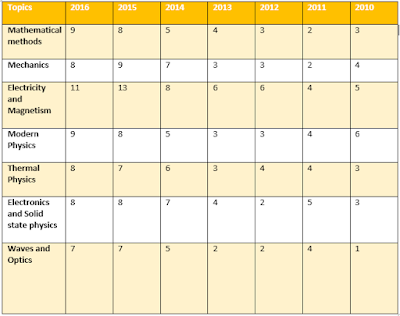Image showing data of all previous year questions

Let's analyse some previous year question papers, because it is very important to know how much gravity a topic carries. So it will help you to make a better strategy for each topic.

As we can see from the above pie chart which are showing that by covering topics electricity and magnetism, mathematical physics,modern physics. mechanics, thermal physics your 74-75% of job is done and if you can do it well then you can crack the exam for sure. To get admission in IITs, a general category candidate need 36-40 marks out of 100. Now you have two choices one is waves and optics and other is electronics. Both are equal important. But I will suggest to go through  waves and optics portion because it has some advantage over electronics portion as the concepts are very familiar to us which we had read in 11th and 12th standard. So it will be unfair to leave it as questions from waves and optics are usually formula based and tricky a little bit.
Quality study material is very important, so I am going suggest some books which I hope, will help you a lot.

### Recommended books for IIT JAM  Physics

 TOPICS BOOKS Mathematical Methods 1.Mathematical Physics by H.K. Das 2.Mathematical methods for physical sciences by M. L. Boas Mechanics & General Properties of Matter 1.An Introduction to Mechanics by Kleppner and Kolenkow 2. Mechanics by D.S. Mathur 3.Concepts of Physics by H.C. Verma 4. Fundamental of physics by halliday       resnick walker Oscillations, Wave and Optics 1. fundamental of physics by halliday resnick     walker 2.Optics by Ajoy Ghatak Electricity and Magnetism 1. Introduction to Electrodynamics by David       J. Griffiths 2. Concepts of physics by H C Verma Kinetic Theory, Thermodynamics 1.Thermodynamics by Garg, Bansal and        Ghosh 2.Heat and thermodynamics by Zemansky Modern physics 1.Fundamental of physics by halliday resnick walker 2. Modern physics by arthur beiser Solid State Physics, Devices and Electronics 1.Electronic devices and circuit theory by Boylestad and Nashelsky 2.Digital Electronics by Malvino and Leach

To check your preparation level, solve previous year question papers and try to improve your weak areas. Solving previous year papers will give you an insight into exam and give you an idea of the trend of the questions.

### Previous year question paper for IIT JAM Physics

*   IIT JAM Physics 2016
*   IIT JAM Physics 2017

### Important dates for IIT JAM (Physics) exam

Commencement of online registration- Fisrt week of September

Date of examination- second Sunday of Feberuary

Announcement of result- Last week of March

### Final tips to prepare Physics for IIT JAM Solve full length mock test paper regularly Topics which carries maximum percentage should be prepared at first Make short notes on each topics and revise them regularly. It is mandatory to solve all the previous year question paper. Share this post to your friends....

How to prepare Physics for IIT JAM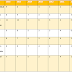Reviewed by Unknown on 00:11 Rating: 5

1.Thank you so much

2.3.Thanks Sir, for giving the preparation tips. I am a B.Sc. final year student and I want to prepare for IIT JAM Physics. I am checked the eligibility criteria from - https://scoop.eduncle.com/iit-jam-eligibility and I found that I am eligible for that exam.
Can you tell me about the new notifications related to IIT JAM 2018.

1.Have You study material for jam ?

2.Thank you so much

4.can i do m.sc astrophysics iit by qualifying iit jam physics...and what is the minimum cutoff for m.sc astrophysics...

5.very good effort.... i realy apreceate you gyes thnk u so much...

6.Thank you very much sir for the guidance:-):-):-)

7.8.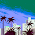1.Dont go too deep into complex number.
In iit jam, only basic knowledhe of complex number is enough.
Different forms of complex
Etc.

9.Great post on how to study physics
thanks for sharing

10.Thanks u very ecelent idea

11.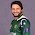Thanks for taking the time to discuss this, I feel strongly about it and love learning more on this topic. If possible, as you gain expertise, would you mind updating your blog with extra information? It is extremely helpful for me. gravity

12.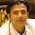Get all education notification with My Next Exam Web Portal

13.Sir I'm preparing for the IIT JAM. When can I fill IIT JAM 2020 application form? What are other eligibility criteria for the exam?